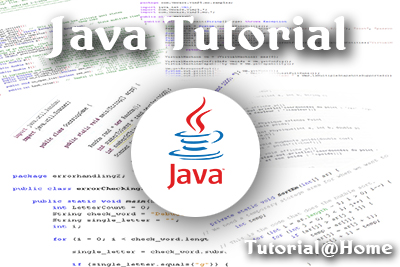FREE ASSISTANCE FOR THE INQUISITIVE PEOPLE
Tutorial Topics
Java String Programs - Replace word in java Actual parameter and Formal parameter
SMITH NUMBERS JAVA - Java
19984    Arnab De    21/04/2018### Check a number is smith number or not.[ISC 2008 Practical]

Defnation: A Smith number is a composite number, the sum of whose digits is the sum of the digits of its prime factors obtained as a result of prime factorization (excluding 1). The first few such numbers are 4, 22, 27, 58, 85, 94, 121 ……………….

121 - 1 + 2 + 1 = 4

prime factors of 121 is 11 11 (excluding 1)

1 + 1 + 1 + 1 = 4

Steps to slove the problems

step 1 : Find the sum of the digits of the given numbers.
step 2 : Find the prime factors.
step 3 : Find the sum of the digits of the prime factors.
step 4 : Compare two result.

import java.util.*;
public class SmithNumbers
{
int sumDigit(int n)
{
int s=0;
while(n>0)
{
s=s+n%10;
n=n/10;
}
return s;
}

int sumPrimeFact(int n)
{
int i=2, sum=0;
while(n>1)
{
if(n%i==0)
{
sum=sum+sumDigit(i);
n=n/i;
}
else
{
do{
i++;
}while(!IsPrime(i));
}
}
return sum;
}
boolean IsPrime(int k)
{
boolean b=true;
int d=2;
while(d<Math.sqrt(k))
{
if(k%d==0)
{
b=false;
}
d++;
}
return b;
}
public static void main(String args[])
{
Scanner sc=new Scanner(System.in);
SmithNumbers ob=new SmithNumbers();
System.out.print("Enter a Number : ");
int n=sc.nextInt();
int a=ob.sumDigit(n);
int b=ob.sumPrimeFact(n);
System.out.println("Sum of Digit = "+a);
System.out.println("Sum of Prime Factor = "+b);
if(a==b)
System.out.print("It is a Smith Number");
else
System.out.print("It is Not a Smith Number");
}
}

Output:
Enter a Number : 85
Sum of Digit = 13
Sum of Prime Factor = 13
It is a Smith Number

Enter a Number : 121
Sum of Digit = 4
Sum of Prime Factor = 4
It is a Smith Number

Enter a Number : 666
Sum of Digit = 18
Sum of Prime Factor = 18
It is a Smith Number

Enter a Number : 999
Sum of Digit = 27
Sum of Prime Factor = 19
It is Not a Smith Number

Java String Programs - Replace word in java Actual parameter and Formal parameter
Author DetailsArnab De
I have over 16 years of experience working as an IT professional, ranging from teaching at my own institute to being a computer faculty at different leading institute across Kolkata. I also work as a web developer and designer, having worked for renowned companies and brand. Through tutorialathome, I wish to share my years of knowledge with the readers.
Enter New Comment
Comment History
No Comment Found Yet.An educated, enlightened and informed population is one of the surest ways of promoting the health of a democracy.
Nelson Mandela
911
46.7
Today So Far
Total View (Lakh)